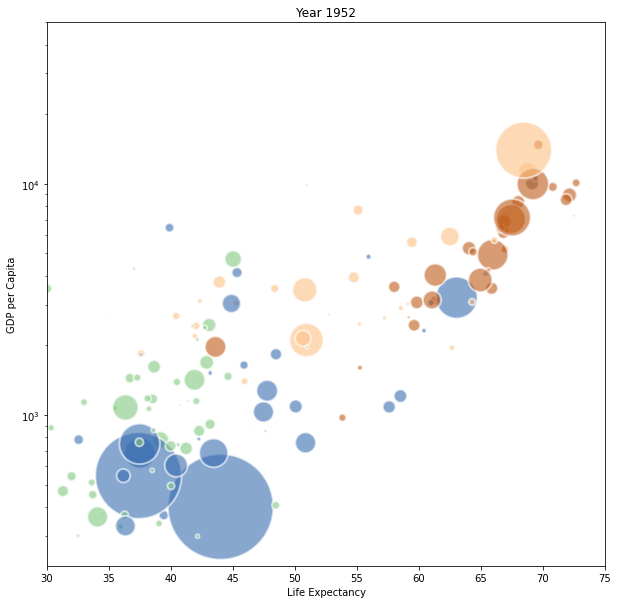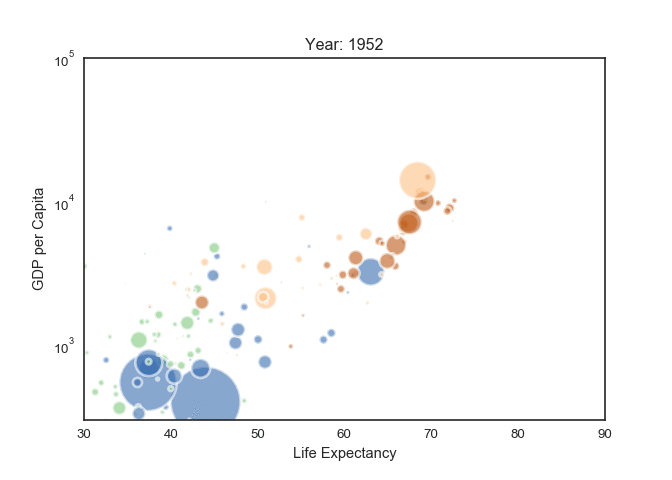# Animated bubble chart

This blogpost explains how to build an animated bubble chart using `Python` and `Image Magick`. `Matplotlib` is used to produce several images, `Image Magick` to concatenate them as a `gif`.

## 📍 Dataset

This example is based on the famous `gapminder` dataset. It provides the average life expectancy, gdp per capita and population size for more than 100 countries. It is available online here and I've stored a copy on the gallery github repo

Let's load it in python and have a look to the 3 first rows.

``````# Libraries
import pandas as pd

# read the data (on the web)

# Check the first 2 rows
country year pop continent lifeExp gdpPercap
0 Afghanistan 1952 8425333.0 Asia 28.801 779.445314
1 Afghanistan 1957 9240934.0 Asia 30.332 820.853030

## 💭 Bubble chart

Let's build a bubble chart for the first year of the dataset (1952). If you're interested in how to make bubble charts with python, the gallery has a dedicated section for it.

Let's build one using the `scatter()` function of `matplotlib`:

``````# import matplotlib
import matplotlib.pyplot as plt

# And I need to transform my categorical column (continent) in a numerical value group1->1, group2->2...
data['continent']=pd.Categorical(data['continent'])

# Set the figure size
plt.figure(figsize=(10, 10))

# Subset of the data for year 1952
data1952 = data[ data.year == 1952 ]

# Scatterplot
plt.scatter(
x = data1952['lifeExp'],
y = data1952['gdpPercap'],
s=data1952['pop']/50000,
c=data1952['continent'].cat.codes,
cmap="Accent",
alpha=0.6,
edgecolors="white",
linewidth=2);

# Add titles (main and on axis)
plt.yscale('log')
plt.xlabel("Life Expectancy")
plt.ylabel("GDP per Capita")
plt.title("Year 1952")
plt.ylim(0,50000)
plt.xlim(30, 75);
``````## 🎥 Animation

An animation is basically a set of static images visualized one after the other. The strategy here is to build on `png` file for each year of the dataset, and then concatenated them as a `gif`.

Let's build a loop that will output one `png` file per year of the dataset:

``````# image resolution
dpi=96

# For each year:
for i in data.year.unique():

# Turn interactive plotting off
plt.ioff()

# initialize a figure
fig = plt.figure(figsize=(680/dpi, 480/dpi), dpi=dpi)

# Find the subset of the dataset for the current year
subsetData = data[ data.year == i ]

# Build the scatterplot
plt.scatter(
x=subsetData['lifeExp'],
y=subsetData['gdpPercap'],
s=subsetData['pop']/200000 ,
c=subsetData['continent'].cat.codes,
cmap="Accent", alpha=0.6, edgecolors="white", linewidth=2)

# Add titles (main and on axis)
plt.yscale('log')
plt.xlabel("Life Expectancy")
plt.ylabel("GDP per Capita")
plt.title("Year: "+str(i) )
plt.ylim(0,100000)
plt.xlim(30, 90)

# Save it & close the figure
filename='/Users/yan.holtz/Desktop/Gapminder_step'+str(i)+'.png'
plt.savefig(fname=filename, dpi=96)
plt.gca()
plt.close(fig)
``````

Now, you should have a set of 12 images in the specified folder (`/Users/yan.holtz/Desktop/` for me). Image magick is a command line tool that allows to concatenate those images in a `gif` file.

Install ImageMagick with this line of bash: `brew install imagemagick`

If you don't have `brew` installed, visit the image magick homepage for explanations.

Once the tool is installed, you can concatenate the 12 images using the following command:

``````# Bash
# convert -delay 80 Gapminder*.png animated_gapminder.gif
``````

Note: the above line of code is written in `bash`, not in `python`. You have to execute it in a terminal, not in your python environment.

And here is the final result## Contact & Edit

👋 This document is a work by Yan Holtz. Any feedback is highly encouraged. You can fill an issue on Github, drop me a message onTwitter, or send an email pasting `yan.holtz.data` with `gmail.com`.